xSylvester's criterionEncyclopedia
In mathematics, Sylvester’s criterion is a necessary and sufficient criterion to determine whether a Hermitian matrix is positive-definite
Positive-definite matrix
In linear algebra, a positive-definite matrix is a matrix that in many ways is analogous to a positive real number. The notion is closely related to a positive-definite symmetric bilinear form ....

. It is named after James Joseph Sylvester
James Joseph Sylvester
James Joseph Sylvester was an English mathematician. He made fundamental contributions to matrix theory, invariant theory, number theory, partition theory and combinatorics...

.

Sylvester's criterion states that a Hermitian matrix M is positive-definite if and only if all the following matrices have a positive determinant
Determinant
In linear algebra, the determinant is a value associated with a square matrix. It can be computed from the entries of the matrix by a specific arithmetic expression, while other ways to determine its value exist as well...

:
• the upper left 1-by-1 corner of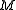,
• the upper left 2-by-2 corner of,
• the upper left 3-by-3 corner of,
• ...
•itself.

In other words, all of the leading principal minors must be positive.

## Proof

The proof is only for nonsingular Hermitian matrix with coefficients in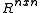, therefore only for nonsingular real-symmetric matrices

Positive Definite or Semidefinite Matrix: A symmetric matrix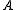whose eigenvalues are positive (λ>0) is called positive definite
Positive-definite matrix
In linear algebra, a positive-definite matrix is a matrix that in many ways is analogous to a positive real number. The notion is closely related to a positive-definite symmetric bilinear form ....

, and when the eigenvalues are just nonnegative (λ≥0),is said to be positive semidefinite.

Theorem I: A real-symmetric matrixhas nonnegative eigenvalues if and only ifcan be factored as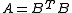, and all eigenvalues are positive if and only if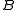is nonsingular.

|-
|valign="top"| Proof: ||
Necessity:
If A ∈ Rnxn is symmetric, then, by the Spectral theorem
Spectral theorem
In mathematics, particularly linear algebra and functional analysis, the spectral theorem is any of a number of results about linear operators or about matrices. In broad terms the spectral theorem provides conditions under which an operator or a matrix can be diagonalized...

, there is an orthogonal matrix P such that A = PDPT , where D = diag (λ1, λ2, . . . , λn) is real diagonal matrix with entries - eigenvalues of A and P is such that it columns are the eigenvectors of A. If λi ≥ 0 for each i, then D1/2 exists, so A = PDPT = PD1/2D1/2PT = BTB for B = D1/2PT, and λi > 0 for each i if and only if B is nonsingular.

Sufficiency:
Conversely, if A can be factored as A = BTB, then all eigenvalues of A are nonnegative because for any eigenpair (λ, x):

λ=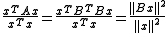≥0.
|}
Theorem II (The Cholesky decomposition): The symmetric matrix A possesses positive pivots if and only if A can be uniquely factored as A = RTR, where R is an upper-triangular matrix with positive diagonal entries. This is known as the Cholesky decomposition
Cholesky decomposition
In linear algebra, the Cholesky decomposition or Cholesky triangle is a decomposition of a Hermitian, positive-definite matrix into the product of a lower triangular matrix and its conjugate transpose. It was discovered by André-Louis Cholesky for real matrices...

of A, and R is called the Cholesky factor of A.
|-
|valign="top"| Proof: ||
Necessity: If A possesses positive pivots (therefore A possesses an LU factorization: A=L.U' ), then, it has an LDU factorization A = LDU=LDLT in which D = diag (u11, u22, . . . , unn) is the diagonal matrix containing the pivots uii > 0.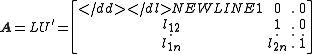x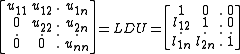x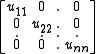x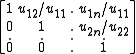By a uniqueness property of the LDU decomposition, the symmetry of A yields: U=LT, consequently A=LDU=LDLT. Setting R = D1/2LT where D1/2 = diag(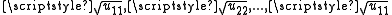) yields the desired factorization, because A = LD1/2D1/2LT = RTR, and R is upper triangular with positive diagonal entries.

Sufficiency: Conversely, if A = RRT , where R is lower triangular with a positive diagonal, then factoring the diagonal entries out of R is as follows: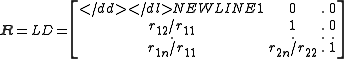x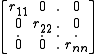R = LD, where L is lower triangular with a unit diagonal and D is the diagonal matrix whose diagonal entries are the rii ’s. Consequently, A = LD2LT is the LDU factorization for A, and thus the pivots must be positive because they are the diagonal entries in D2.
|}
Theorem III: Let Ak be the k × k leading principal submatrix of An×n. If A has an LU factorization A = LU, then det(Ak) = u11u22 · · · ukk, and the k-th pivot is ukk =det(A1) = a11 for k = 1, ukk=det(Ak)/det(Ak−1) for k = 2, 3, . . . , n.

Combining Theorem II with Theorem III yields:

Statement I: If the symmetric matrix A can be factored as A=RTR where R is an upper-triangular matrix with positive diagonal entries, then all the pivots of A are positive (by Theorem II), therefore all the leading principal minors of A are positive (by Theorem III).

Statement II: If the nonsingular symmetric matrix A can be factored as, then the QR decomposition
QR decomposition
In linear algebra, a QR decomposition of a matrix is a decomposition of a matrix A into a product A=QR of an orthogonal matrix Q and an upper triangular matrix R...

(closely related to Gram-Schmidt process) of B (B=QR) yields: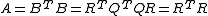, where Q is orthogonal matrix
Orthogonal matrix
In linear algebra, an orthogonal matrix , is a square matrix with real entries whose columns and rows are orthogonal unit vectors ....

and R is upper triangular matrix
Triangular matrix
In the mathematical discipline of linear algebra, a triangular matrix is a special kind of square matrix where either all the entries below or all the entries above the main diagonal are zero...

.

Namely Statement II requires the non-singularity of the symmetric matrix A.

Combining Theorem I with Statement I and Statement II yields:

Statement III: If the real-symmetric matrix A is positive definite then A possess factorization of the form A=BTB, where B is nonsingular (Theorem I), the expression A=BTB implies that A possess factorization of the form A=RTR where R is an upper-triangular matrix with positive diagonal entries (Statement II), therefore all the leading principal minors of A are positive (Statement I).

In other words, Statement III states:

Sylvester's Criterion: The real-symmetric matrix A is positive definite if and only if all the leading principal minors of A are positive.

The sufficiency and necessity conditions automatically holds because they were proven for each of the above theorems.